##### Pre-Calculus For Dummies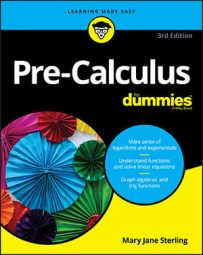When you’re given a problem in radical form, you may have an easier time if you rewrite it by using rational exponents — exponents that are fractions. You can rewrite every radical as an exponent by using the following property — the top number in the resulting rational exponent tells you the power, and the bottom number tells you the root you’re taking: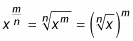For example, you can rewrite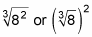as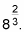Fractional exponents are roots and nothing else. For example, 641/3 doesn’t mean 64–3 or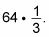In this example, you find the root shown in the denominator (the cube root) and then take it to the power in the numerator (the first power). So 641/3 = 4.

The order of these processes really doesn’t matter. You can choose either method:

• Cube root the 8 and then square that product

• Square the 8 and then cube root that product

Either way, the equation simplifies to 4. Depending on the original expression, though, you may find the problem easier if you take the root first and then take the power, or you may want to take the power first. For example, 643/2 is easier if you write it as (641/2)3 = 83 = 512 rather than (643)1/2, because then you’d have to find the square root of 262,144.

Take a look at some steps that illustrate this process. To simplify the expression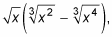rather than work with the roots, execute the following:

1. Rewrite the entire expression using rational exponents.

Now you have all the properties of exponents available to help you to simplify the expression: x1/2(x2/3x4/3).

2. Distribute to get rid of the parentheses.

When you multiply monomials with the same base, you add the exponents.

Hence, the exponent on the first term is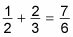and the exponent of the second term is 1/2+4/3=11/6. So you get x7/6x11/6.

3. Because the solution is written in exponential form and not in radical form, as the original expression was, rewrite it to match the original expression.

This gives you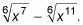Typically, your final answer should be in the same format as the original problem; if the original problem is in radical form, your answer should be in radical form. And if the original problem is in exponential form with rational exponents, your solution should be as well.